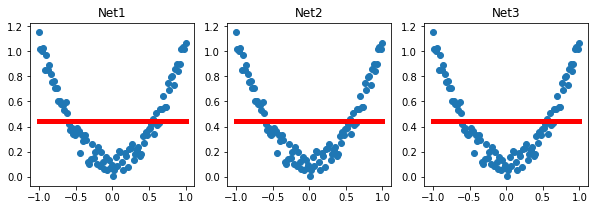How to save and reload a deep learning model in Pytorch?
MACHINE LEARNING RECIPES DATA CLEANING PYTHON DATA MUNGING PANDAS CHEATSHEET     ALL TAGS

# How to save and reload a deep learning model in Pytorch?

This Pytorch recipe provides you a solution for saving and loading Pytorch models - entire models or just the parameters.

3

This recipe provides options to save and reload an entire model or just the parameters of the model. While reloading this recipe copies the parameter from 1 net to another net. There are 3 main functions involved in saving and loading a model in pytorch.

1. torch.save: This saves a serialized object to disk. It uses python's pickle utility for serialization. Models, tensors and dictionaries can be saved using this function.
2. torch.load: torch.load: Uses pickle's unpickling facilities to deserialize pickled object files to memory. This function also facilitates the device to load the data into.
3. torch.nn.Module.load_state_dict: Loads a model's parameter dictionary using a deserialized state_dict. The learnable parameters (i.e. weights and biases) of an torch.nn.Module model are contained in the model's parameters (accessed with model.parameters()). A state_dict is simply a Python dictionary object that maps each layer to its parameter tensor.

What is PyTorch ?
Pytorch is a Python-based scientific computing package that uses the power of graphics processing units and can replace the numpy library. It is also a very popular deep learning research platform built for flexibility and speed. You can use other Python packages such as NumPy, SciPy to extend PyTorch functionalities.

What is Deep Learning Model ?
Deep learning is a subset of machine learning. Deep learning uses neural networks to make predictions. A neural network takes inputs, which are then processed using hidden layers using weights that are adjusted during training. The model then outputs a prediction.

In :
```import torch
import matplotlib.pyplot as plt
%matplotlib inline

torch.manual_seed(1)    # reproducible
```
Out:
`<torch._C.Generator at 0x12214f310>`
In :
```#sample data
x = torch.unsqueeze(torch.linspace(-1, 1, 100), dim=1)  # x data (tensor), shape=(100, 1)
y = x.pow(2) + 0.2*torch.rand(x.size())  # noisy y data (tensor), shape=(100, 1)
```
In :
```def save():
# save net1
net1 = torch.nn.Sequential(
torch.nn.Linear(1, 10),
torch.nn.ReLU(),
torch.nn.Linear(10, 1)
)
optimizer = torch.optim.SGD(net1.parameters(), lr=0.5)
loss_func = torch.nn.MSELoss()

for t in range(100):
prediction = net1(x)
loss = loss_func(prediction, y)
loss.backward()
optimizer.step()

# plot result
plt.figure(1, figsize=(10, 3))
plt.subplot(131)
plt.title('Net1')
plt.scatter(x.data.numpy(), y.data.numpy())
plt.plot(x.data.numpy(), prediction.data.numpy(), 'r-', lw=5)

# 2 ways to save the net
torch.save(net1, 'net.pkl')  # save entire net
torch.save(net1.state_dict(), 'net_params.pkl')   # save only the parameters
```
In :
```def restore_net():
# restore entire net1 to net2
prediction = net2(x)

# plot result
plt.subplot(132)
plt.title('Net2')
plt.scatter(x.data.numpy(), y.data.numpy())
plt.plot(x.data.numpy(), prediction.data.numpy(), 'r-', lw=5)
```
In :
```def restore_params():
# restore only the parameters in net1 to net3
net3 = torch.nn.Sequential(
torch.nn.Linear(1, 10),
torch.nn.ReLU(),
torch.nn.Linear(10, 1)
)

# copy net1's parameters into net3
prediction = net3(x)

# plot result
plt.subplot(133)
plt.title('Net3')
plt.scatter(x.data.numpy(), y.data.numpy())
plt.plot(x.data.numpy(), prediction.data.numpy(), 'r-', lw=5)
plt.show()
```
In :
```# save net1
save()
# restore entire net (may slow)
restore_net()
# restore only the net parameters
restore_params()
```#### Relevant Projects

##### Predict Macro Economic Trends using Kaggle Financial Dataset
In this machine learning project, you will uncover the predictive value in an uncertain world by using various artificial intelligence, machine learning, advanced regression and feature transformation techniques.

##### PySpark Tutorial - Learn to use Apache Spark with Python
PySpark Project-Get a handle on using Python with Spark through this hands-on data processing spark python tutorial.

##### Predict Credit Default | Give Me Some Credit Kaggle
In this data science project, you will predict borrowers chance of defaulting on credit loans by building a credit score prediction model.

##### Data Science Project-TalkingData AdTracking Fraud Detection
Machine Learning Project in R-Detect fraudulent click traffic for mobile app ads using R data science programming language.

##### Build an Image Classifier for Plant Species Identification
In this machine learning project, we will use binary leaf images and extracted features, including shape, margin, and texture to accurately identify plant species using different benchmark classification techniques.

##### Data Science Project on Wine Quality Prediction in R
In this R data science project, we will explore wine dataset to assess red wine quality. The objective of this data science project is to explore which chemical properties will influence the quality of red wines.

##### Music Recommendation System Project using Python and R
Machine Learning Project - Work with KKBOX's Music Recommendation System dataset to build the best music recommendation engine.

##### Predict Churn for a Telecom company using Logistic Regression
Machine Learning Project in R- Predict the customer churn of telecom sector and find out the key drivers that lead to churn. Learn how the logistic regression model using R can be used to identify the customer churn in telecom dataset.

##### Predict Employee Computer Access Needs in Python
Data Science Project in Python- Given his or her job role, predict employee access needs using amazon employee database.

##### Sequence Classification with LSTM RNN in Python with Keras
In this project, we are going to work on Sequence to Sequence Prediction using IMDB Movie Review Dataset​ using Keras in Python.